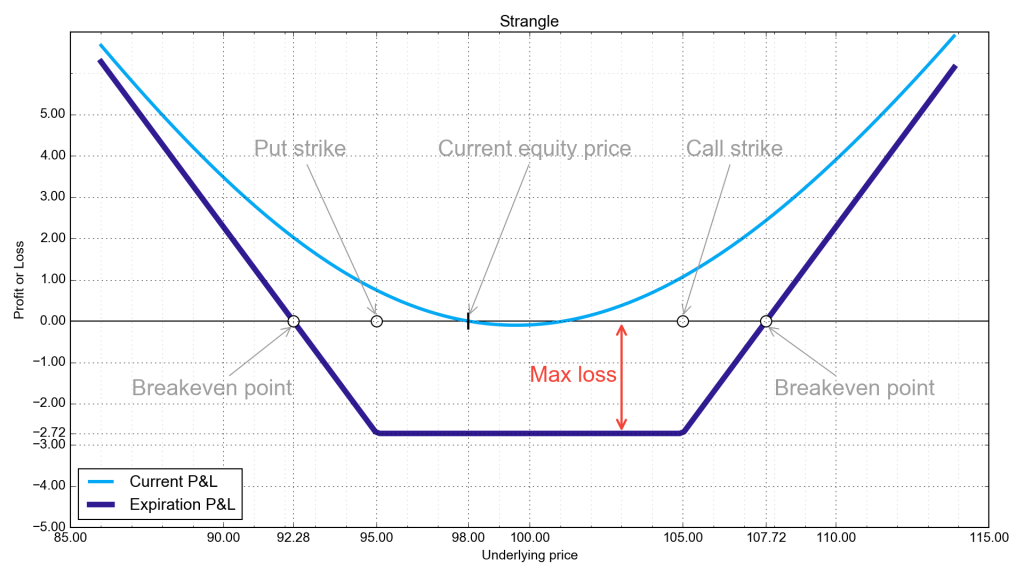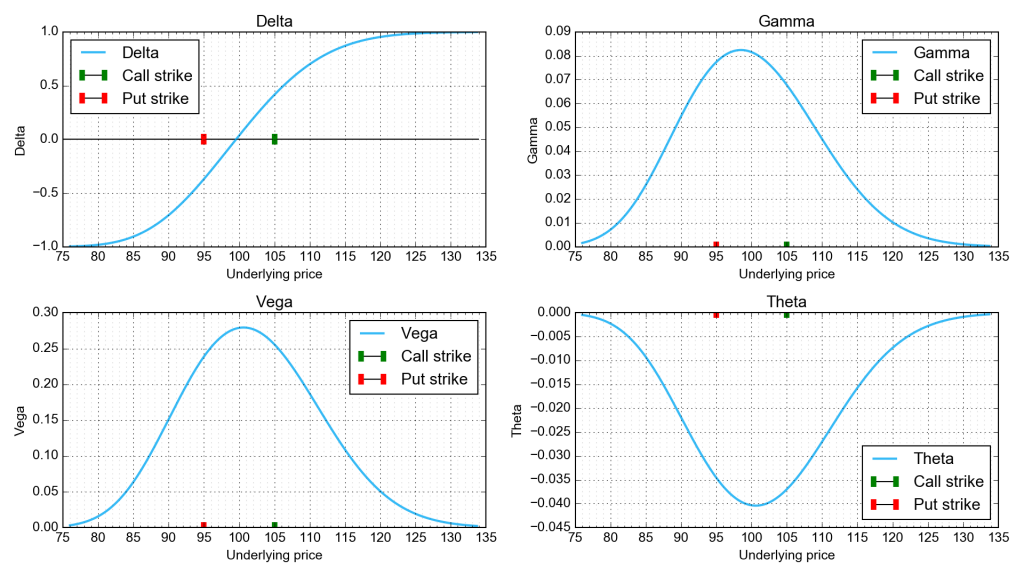# Strangle

## Strategy name and alternative names

Strangle. Alternative shorter names are Long strangle, poor-man’s straddle.

## Main characteristics

Neutral position. It is a combination involving an equal number of out-of-the-money (`OTM`) long puts and long calls with the same expiration date. It is a debit combination, which means you must pay to put on the position. The strategy profits when the price of the underlying security moves up or down beyond the breakeven points.

## Options used in the combination

Buy to open one OTM call and simultaneously buy to open one OTM put. Both options derive from the same underlying stock. The strike price of the put is below the current stock price by about the same amount as the call strike price is above the security price. For example, if the stock price is `100`, you would buy a 95-strike put and a 105-strike call. The advantage of this combination is that it benefits from volatility, independently of the direction of stock price movement. Both the put and the call have (potentially) unlimited upsides but limited loss exposure.
A strangle is like a straddle, except that the put and call in a straddle have the same at-the-money strike price. Because the strangle uses cheaper OTM options, the total premium is less than that for a straddle, all other things being equal.

## Risk profileMaximum profit : If the stock price rises above the call’s strike, the profit on the call leg is unlimited, and is equal to the difference between the underlying’s price and the call strike price, minus the premium on the strangle (we’ll ignore commissions). Upside potential on the call leg is unlimited. If the stock price falls, the profit on the put leg is virtually unlimited, and is equal to the difference between the put strike price and the underlying’s price, minus the premium on the strangle. (Technically, profit potential on the put leg is limited to the strike price of the put minus the premium, because a stock price can drop to no lower than zero.)

Maximum loss : Premium paid, which equals the premium on the call plus the premium on the put. Occurs when the underlying’s price closes between the call and put strikes.

Breakeven point : There are two breakeven points on a strangle. The upper breakeven point equals the call strike price plus the total premium paid. The lower breakeven point equals the put strike price minus the total premium paid.

Key points : On a profit/loss chart where the X axis is a base asset price and the Y axis is profit/loss, the value of the combination is a minimum between the put strike price and the call strike price. The two strike prices form the base of a truncated V-shaped curve, that is, two diagonal lines with opposite slopes that rise from the endpoints of the line connecting the put and call strike prices. The left leg begins at a point with (X,Y) coordinates of (put strike price, stock price minus strangle premium). The right leg begins at a point with (X,Y) coordinates of (call strike price, stock price minus strangle premium). The maximum loss, at any point between the two strike prices, is the strangle premium. The left (put) leg rises as the stock price falls and is bounded by the y-axis. The right (call) leg rises without limit as the stock price rises. The left and right legs cross the X axis at the breakeven points described above.

## Preconditions to open this combination

You would put on a strangle in the hopes that the underlying stock will quickly break out above the upper breakeven point or break down below the lower breakeven point. You would choose an underlying stock that is relatively volatile, as this increases the chances that the stock price will move away from the strike prices (see the vega chart). You don’t need an opinion on price direction to profit from a strangle, as long as the stock price moves a sufficient amount. Some traders put on strangles just ahead of a corporate announcement, jury verdict or earnings announcement, in the hopes the news will move the stock price. Your biggest fear is that the price of the underlying stock will not move into the money enough (for either the put or the call) to recoup all your premium.

## Greeks### Delta

Delta is an option’s probable price movement in relation to the price movement of the underlying stock. The value ranges from `-1` to `1`:

• `-1` to `0` for a long put;
• `0` to `1` for a long call;

where `0` means the option (and thus the spread) price is not affected by the stock price, and `1` means the option prices moves penny for penny in lockstep with the underlying’s price. At the average strike price of a strangle, the deltas of the put and of the call cancel each other out, which is why the strangle is delta neutral. There is a wrinkle when the stock price does not exactly match the average strike price. If the stock price is above the average strike price, the call will have a slightly higher delta value than will the put, giving you a positive delta position that, in the short term, gains on the upside and loses to the downside. The reverse is true if the stock price is below the average strike price. This is only a concern if you sell to close the strangle before expiration; If you hold till expiration, the effect of a slightly non-neutral delta evaporates.

### Vega

Vega is the effect of the underlying stock’s volatility on the option’s price. For a strangle, higher vega translates into higher option prices, all things being equal. For example, a vega of `0.3` means that an option’s price will rise by 30 cents for each point increase in the underlying stock’s implied volatility. Buying a strangle during periods of high implied volatility can be disadvantageous, because a volatility crunch will shrink vega and collapse the time value of the put and call premiums. Vega has a maximum value between strikes.

### Theta

Theta is the time decay of an option’s price as the expiration date approaches. At the close of expiration day, the option’s theta is `0`. Theta is lower for long expiration periods, which means the option loses time value more slowly than it would for short expiration periods. A longer expiration period increases the chance that the combination will expire in the money. Theta for a strangle is negative (which means the combination loses value over time) because the options lose value due to time decay. Time decay speed is maximum when the underlying’s price is between strikes.

### Gamma

Gamma is the rate of change of delta – the responsiveness of the position to the underlying’s price movement. In a strangle, gamma is positive, which means delta will increase in the direction of the stock price movement. On an upward stock move, a positive gamma dictates that the delta of the strangle becomes increasingly positive, while on a downward stock move, the strangle’s delta becomes increasingly negative. You can thus profit from a move in either direction with a strangle, as long as the stock moves far enough into the money for either the put or the call.

## Position example

Assume its mid-January and United Technologies (UTX) is trading at `\$110.00`. Buy to open 10 UTX March 03 114-strike calls for `\$1.12` each, buy to open 10 UTX March 03 106-strike puts for `\$1.23 each`. Neglect commissions.

• Net Debit: `\$1.12 + \$1.23 = \$2.35`. You pay `\$2,350`.
• Maximum profit: `10 x (|ITM option strike price – stock price| – \$235)`
• Maximum loss: `\$2,350`.
• Breakeven point 1: `\$114 + \$2.35 = \$116.35`.
• Breakeven point 2: `\$106 – \$2.35 = \$103.65`.

## How to manage position

This is a debit combination with limited risk and unlimited reward. Here are some things you can do.

### Strong move in underlying stock price

1. You can sell to close the leg that gained value due to the move in the underlying stock price. If time decay hasn’t devalued options significantly, the option in the favored leg has become profitable. Alternatively, you can sell the whole spread,
2. You can sell to open an option one to two strikes further in the money than the option that gained value. Doing this converts one side of the strangle into a vertical spread, thus compensating you for the premium you paid on the strangle, possibly creating a positive risk profile.
3. If the underlying stock moves beyond one of the breakeven points, you can sell to close the out-of-the-money (OTM) option to recoup a little of the premium.

### Strong move in underlying stock price that you expect to continue

You could sell to close the OTM option and use the proceeds to buy more contracts of the in-the-money option, at a strike price of your choice.

## Variations

If you wish to risk more money in order to reduce the distance between the two breakeven points, you could buy a straddle instead of a strangle.

If you have a mildly bullish inclination, you could buy more calls than puts. If you have a mildly bearish inclination, you could buy more put than calls. You’ll make more profit with either variation than you would with a classic strangle if you correctly guess the direction of stock movement, but you’ll profit less (or lose more) if you guess incorrectly.

## Summary

A strangle limits your losses to some amount between zero and the combination’s premium if the stock price remains between the two breakeven points. There is no profit limit on the upside, and the downside profit is limited only to the extent that a stock price can’t fall below zero. If the stock price moves substantially, your ROI will be smaller than it would be from a naked long call or put position, but you would have to correctly guess the direction of the movement to realize the higher ROI. You might be able to make a profit or cut your loss on a strangle even if the stock price doesn’t move beyond a breakeven point. This is possible only when the implied volatility of the underlying stock jumps soon after purchase and thereby expands option premiums (through an increase in time value) above the amount you paid. You could then sell the strangle for a profit, albeit probably a small profit.

A strangle costs less than a straddle, so that less money is at risk per combination. However, the strangle has a larger distance between breakeven points, so that you need more stock price movement to make a profit. The strangle expires without value if the ending stock price is between the two strike prices, inclusively. In a straddle, the expiration value is more than zero unless the ending stock price is equal to the strike price.